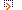# Math DirectoryFeatured Listings

Math Help Forum
Math Help Forum is a free math forum for maths help and answers to mathematics questions of all levels.
Keywords: math forum , math help , math help forum , math homework help , maths forum
www.mathhelpforum.com/math-help/

Math Forum
Math Help: math forum, math problems, mathematical journal, articles, Weekly Problem, math links, etc ...
Keywords: analysis , equalities , formulas , forum , galois , math , mathematics , number
www.mymathforum.com/

Math Is Fun Forum
A discussion forum for K-12 math students and teachers, with a focus on learning and teaching math the fun way.
www.mathisfunforum.com/

Site Listings

Math Help Forum
Math Help Forum is a free math forum for maths help and answers to mathematics questions of all levels.
Keywords: math forum , math help , math help forum , math homework help , maths forum
www.mathhelpforum.com/math-help/

Math Forum
Math Help: math forum, math problems, mathematical journal, articles, Weekly Problem, math links, etc ...
Keywords: analysis , equalities , formulas , forum , galois , math , mathematics , number
www.mymathforum.com/

Math Is Fun Forum
A discussion forum for K-12 math students and teachers, with a focus on learning and teaching math the fun way.
www.mathisfunforum.com/

Add Url or Add Site to Submit Site to the Math Directory